# A more accurate Tline model

New for August 2020. Have you ever been frustrated by the lack of ability to model dielectric loss-tangent loss and substrate conductivity loss in a linear "Tline" element, such as "TLINP" in Microwave Office? That model can take into account metal loss only, by assigning a frequency and scaling attenuation as square-root of frequency. What if you wanted to include dielectric loss (proportional to frequency) or substrate conductivity loss (constant with frequency)? Here's how to do it.

First, note that the variable _FREQ is assigned to the current frequency value in a sweep, so you can do some simple math. Then you have to "pre-scale" the dielectric and conductivity losses. Below, we have assigned 10 dB/meter at 10 GHz to all three types of losses.  Then, dielectric loss is multiplied by square-root of frequency, and substrate conductivity loss is divided by square-root of frequency. Trust us, it all works out in the end...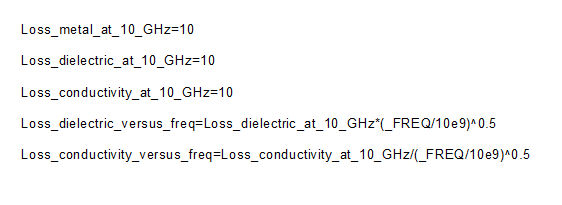Note that Keysight's ADS already has the ability to distinguish between these three losses in their "TLINP" model. Maybe you get what you pay for?

In the model below, we have assigned metal loss to a transmission line between ports 1 and 2, loss tangent loss between ports 3 and 4, conductivity loss between ports 5 and 6, and a composite of all three between ports 7 and 8. Note that we scaled the loss down to one centimeter (10,000um) as loss per meter tends to scare marketing people, managers and customers.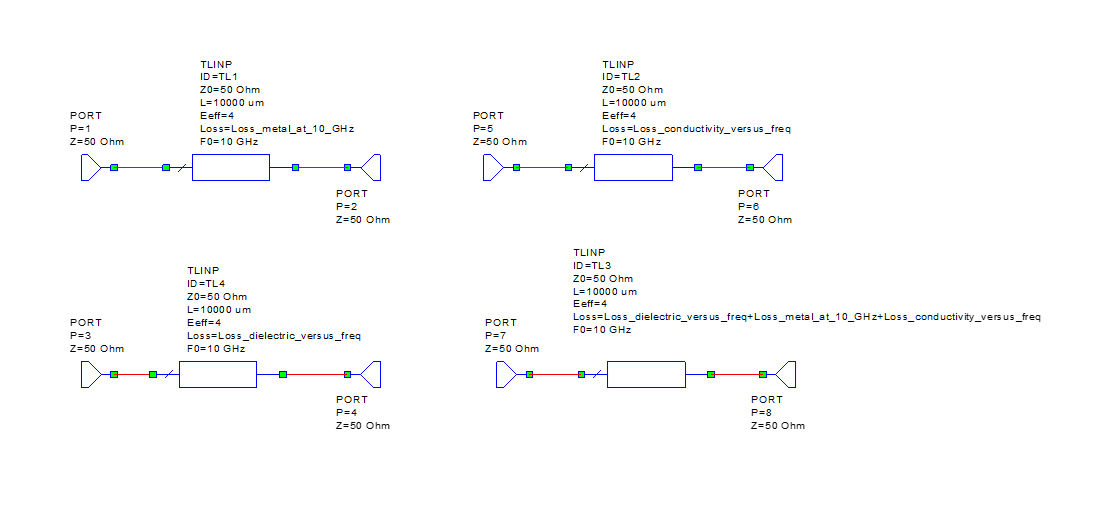Now let's look at the four plots: S21 shows a perfect square-root-of-frequency curve for metal loss, with 0.1 dB/cm at 10 GHz.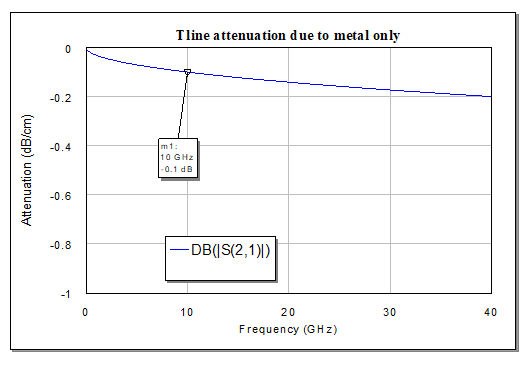S43 shows a perfect linear curve for loss tangent loss, with 0.1 dB/cm at 10 GHz.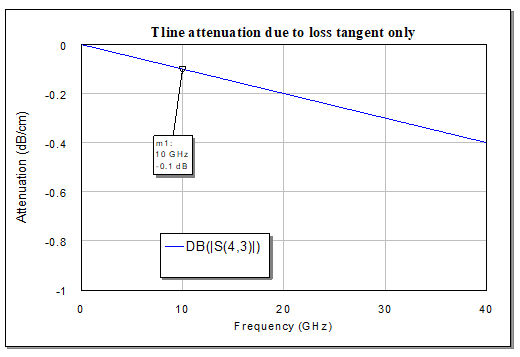S65 shows constant loss associated with substrate conductivity, with 0.1 dB/cm at all frequencies.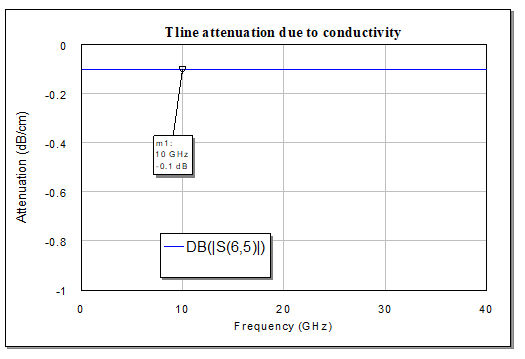And S87 shows the composite response, with 0.3 dB/cm at 10 GHz.  It all adds up!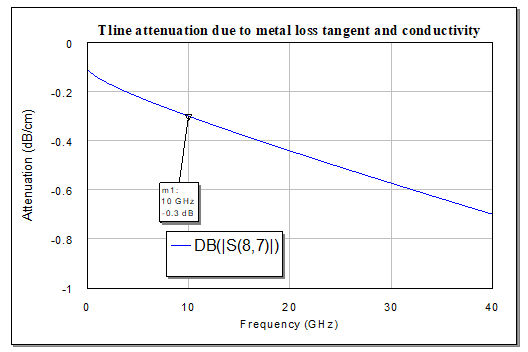Why would you ever want to do this? Suppose you were doing some math-based design, like a traveling-wave combiner, and you wanted an accurate accounting of the loss without converting the ideal elements to physical elements like stripline or microstrip? This technique also helps if you are trying to back-fit measured S-parameters to a linear model, you can let the three loss mechanisms optimize to help fit the results.

Author : Unknown Editor